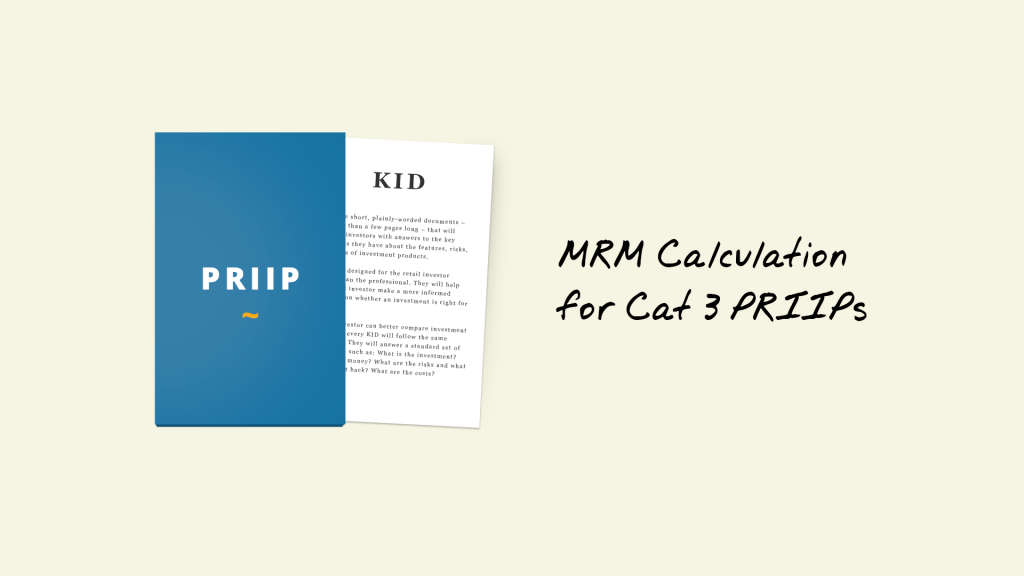In one of our last blog posts we explained details on the Market Risk Measure (MRM) Calculation for Category 2 PRIIPs. Category 2 PRIIPs include standard unit linear products. Those products offer non-leveraged exposure, or leveraged exposure, but with constant multiples. In contrast, Category 3 PRIIPs reflect the prices of underlying investments that are not reflected as a constant multiple. This includes e.g. structured products with caps and/or floors. For those PRIIPs the Regulatory Technical Standards (RTS) published in April 2017 by the European Commission include different calculation methods for the Market Risk Measure (MRM) which is, next to the Credit Risk Measure (CRM), needed to define the Summary Risk Indicator (SRI).

While the MRM calculation for Cat 2 PRIIPs is based on a modified Value at Risk (VaR) of the historical returns of the PRIIP itself, the methodology for Cat 3 PRIIPs is based on simulating the product’s underlying(s). This calculation requires at least 10.000 simulations of the value of those underlyings where 5 years of historical market data is considered (or at least 2 years of daily or 4 years of weekly prices).

Before starting the simulation it is important to differentiate between 2 different types of underlyings. The pay-off of the PRIIP can either depend on curves or on spot prices (or levels).

## Curves

For curves a Principal Component Analysis (PCA) needs to be performed to ensure that the simulation results in a consistent curve. After ensuring that each tenor point is positive, the PCA includes the calculation of the covariance matrix on the basis of continuous returns of the historical tenor points. The eigenvectors with the three largest eigenvalues are selected for the calculation of the matrix of returns that will be used in the simulation. As continuous returns are additive, the returns for each tenor point can be summed up using the matrix of returns. As the returns are calculated using the logarithm, the exponential of the simulated return needs to be multiplied by the current rate at each tenor point to receive the simulated rate. The simulation comprises at least 10.000 iterations for each tenor point until the end of the recommended holding period.

## Spot prices (or levels)

For spot prices the returns are also calculated using the logarithm. The continuous returns need to be calculated for every period in the historical data set before one observed period corresponding to the return of all underlying contracts is randomly picked (bootstrapping methodology). For each contract the simulated return at the recommended holding period can be calculated additively using several bootstrapping runs including a correction for risk neutrality, the impact of the mean of the observed returns as well as the impact of the variance of the observed returns. In case the underlying is denoted in a different currency there is a last correction factor based on the correlation between the asset price and the relevant FX rate measured over the recommended holding period.The price of each underlying can be calculated using the exponential of the return. For each underlying price or level in the historical data set, the simulation comprises at least 10.000 iterations (same as for each tenor point for curves).

Independently from the type of underlying, the resulting 10.000 or more simulated values need to be sorted, which allows for selecting the “simulated empirical VaR” at the 97.5% interval. The VaR is needed for the calculation of the VaR-equivalent volatility (VEV) using the following formula:In case you already read our blog post about the MRM Calculation for Cat 2 PRIIPs, you may have noticed that this formula looks familiar. This is caused by the fact, that both formulas use the same model for the calculation of the VEV. The only difference is that for Cat 2 the formula is based on the return space while for Cat 3 we are calculating the VEV using prices. The assignment of the Market Risk Measure (MRM) class based on the VEV follows also the same rules for Cat 2 and Cat 3 PRIIPs.